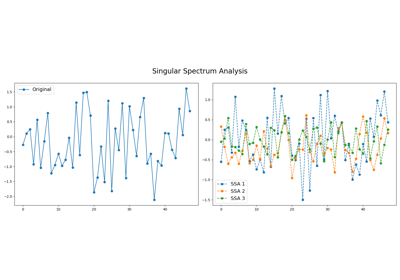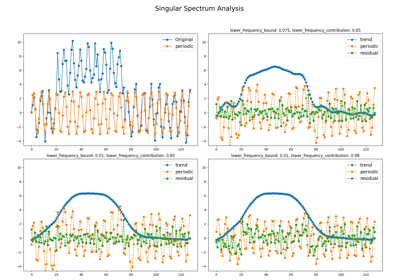# `pyts.decomposition`.SingularSpectrumAnalysis¶

class `pyts.decomposition.``SingularSpectrumAnalysis`(window_size=4, groups=None, lower_frequency_bound=0.075, lower_frequency_contribution=0.85, chunksize=None, n_jobs=1)[source]

Singular Spectrum Analysis.

Parameters: window_size : int or float (default = 4) Size of the sliding window (i.e. the size of each word). If float, it represents the percentage of the size of each time series and must be between 0 and 1. The window size will be computed as `max(2, ceil(window_size * n_timestamps))`. groups : None, int, ‘auto’, or array-like (default = None) The way the elementary matrices are grouped. If None, no grouping is performed. If an integer, it represents the number of groups and the bounds of the groups are computed as `np.linspace(0, window_size, groups + 1).astype('int64')`. If ‘auto’, then three groups are determined, containing trend, seasonal, and residual. If array-like, each element must be array-like and contain the indices for each group. lower_frequency_bound : float (default = 0.075) The boundary of the periodogram to characterize trend, seasonal and residual components. It must be between 0 and 0.5. Ignored if `groups` is not set to ‘auto’. lower_frequency_contribution : float (default = 0.85) The relative threshold to characterize trend, seasonal and residual components by considering the periodogram. It must be between 0 and 1. Ignored if `groups` is not set to ‘auto’. chunksize : int or None (default = None) If int, the transformation of the whole dataset is performed using chunks (batches) and `chunksize` corresponds to the maximum size of each chunk (batch). If None, the transformation is performed on the whole dataset at once. Performing the transformation with chunks is likely to be a bit slower but requires less memory. n_jobs : None or int (default = None) The number of jobs to use for the computation. Only used if `chunksize` is set to an integer.

References

  N. Golyandina, and A. Zhigljavsky, “Singular Spectrum Analysis for Time Series”. Springer-Verlag Berlin Heidelberg (2013).
  T. Alexandrov, “A Method of Trend Extraction Using Singular Spectrum Analysis”, REVSTAT (2008).

Examples

```>>> from pyts.datasets import load_gunpoint
>>> from pyts.decomposition import SingularSpectrumAnalysis
>>> X, _, _, _ = load_gunpoint(return_X_y=True)
>>> transformer = SingularSpectrumAnalysis(window_size=5)
>>> X_new = transformer.transform(X)
>>> X_new.shape
(50, 5, 150)
```

Methods

 `__init__`([window_size, groups, …]) Initialize self. `fit`([X, y]) Pass. `fit_transform`(X[, y]) Fit to data, then transform it. `get_params`([deep]) Get parameters for this estimator. `set_params`(**params) Set the parameters of this estimator. `transform`(X) Transform the provided data.
`__init__`(window_size=4, groups=None, lower_frequency_bound=0.075, lower_frequency_contribution=0.85, chunksize=None, n_jobs=1)[source]

Initialize self. See help(type(self)) for accurate signature.

`fit`(X=None, y=None)[source]

Pass.

Parameters: X ignored y Ignored self : object
`fit_transform`(X, y=None, **fit_params)

Fit to data, then transform it.

Fits transformer to X and y with optional parameters fit_params and returns a transformed version of X.

Parameters: X : array-like, shape = (n_samples, n_timestamps) Univariate time series. y : None or array-like, shape = (n_samples,) (default = None) Target values (None for unsupervised transformations). **fit_params : dict Additional fit parameters. X_new : array Transformed array.
`get_params`(deep=True)

Get parameters for this estimator.

Parameters: deep : bool, default=True If True, will return the parameters for this estimator and contained subobjects that are estimators. params : dict Parameter names mapped to their values.
`set_params`(**params)

Set the parameters of this estimator.

The method works on simple estimators as well as on nested objects (such as `Pipeline`). The latter have parameters of the form `<component>__<parameter>` so that it’s possible to update each component of a nested object.

Parameters: **params : dict Estimator parameters. self : estimator instance Estimator instance.
`transform`(X)[source]

Transform the provided data.

Parameters: X : array-like, shape = (n_samples, n_timestamps) X_new : array-like, shape = (n_samples, n_splits, n_timestamps) Transformed data. `n_splits` value depends on the value of `groups`. If `groups=None`, `n_splits` is equal to `window_size`. If `groups` is an integer, `n_splits` is equal to `groups`. If `groups='auto'`, `n_splits` is equal to three. If `groups` is array-like, `n_splits` is equal to the length of `groups`. If `n_splits=1`, `X_new` is squeezed and its shape is (n_samples, n_timestamps).

## Examples using `pyts.decomposition.SingularSpectrumAnalysis`¶Singular Spectrum AnalysisTrend-Seasonal decomposition with Singular Spectrum Analysis